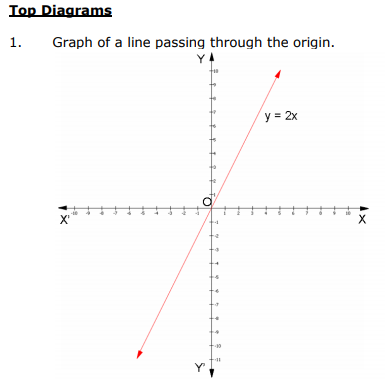# CBSE Class 9 Mathematics Linear Equations In Two Variables Notes Set B

CBSE Class 9 Concepts for Linear Equations in Two Variables (1). Learning the important concepts is very important for every student to get better marks in examinations. The concepts should be clear which will help in faster learning. The attached concepts made as per NCERT and CBSE pattern will help the student to understand the chapter and score better marks in the examinations.

Chapter 4:

Linear Equations in Two Variables

Chapter Notes

Top Definitions

1. An equation of the form ax + by + c = 0, where a, b and c are real numbers, such that a and b are not both zero, is called a linear equation in two variables.

2. A linear equation in two variables is represented geometrically by a straight line the points of which make up the collection of solutions of equation. This is called the graph of the linear equation.

Top Concepts

1. A linear equation in two variables has infinitely many solutions.

2. The graph of every linear equation in two variables is a straight line.

3. x = 0 is the equation of the y – axis and y = 0 is the equation of the x–axis.

4. The graph of x = k is a straight line parallel to the y –axis.

5. The graph of y = k is a straight line parallel to the x – axis.

6. An equation of the type y = mx represents a line passing through the origin, where m is a real number.

7. Every point on the line satisfies the equation of the line and every solution of the equation is a point on the line.

8. The solution of a linear equation is not effected when:

(i) The same number is added or subtracted from both the side of an equation.

(ii) Multiplying or dividing both the sides of the equation by the same non zero number.## Tags:

Click for more Linear Equations in two variables Study Material

## Latest NCERT & CBSE News

Read the latest news and announcements from NCERT and CBSE below. Important updates relating to your studies which will help you to keep yourself updated with latest happenings in school level education. Keep yourself updated with all latest news and also read articles from teachers which will help you to improve your studies, increase motivation level and promote faster learning

### Training Programme on the Alternative Academic Calendar

In view of the extraordinary situation prevailing in the world due to COVID-19 pandemic, the CBSE Board had announced the ‘Revised Academic Curriculum for classes 9- 12 for the session 2020-21’. The reduced topics will not be part of the internal assessment or for the...

### CBSE Reduced Syllabus Class 9 and 10

The prevailing health emergency in the country and at different parts of the world as well as the efforts to contain the spread of Covid-19 pandemic has resulted in loss of class room teaching due to closure of schools. Therefore the CBSE Board has decided to revise...

### FAQs CBSE Board Exam Results Class 10 and 12

FAQs CBSE Board Exam Results Class 10 and 12 Q.1. What does the term RT in the marksheet mean? Ans.The term RT means REPEAT IN THEORY. This is the term used from 2020 instead of FAIL IN THEORY(FT) Q.2. What does the term RP in the marksheet mean? Ans.The term RP means...

### Board Cancelled Official CBSE Statement

Keeping in view the requests received from various State Governments and the changed circumstances as on date, following has been decided- 1. Examinations for classes X and XII which were scheduled from 1st July to 15th, 2020 stand cancelled. 2. Assessment of the...

### Re verification of Class 10 Board Exams Marks

Modalities and Schedule for Secondary School (Class X) Examinations, 2020 in the subjects whose examinations have been conducted by CBSE for the processes of (I) Verification of Marks (II) Obtaining Photocopy of the Evaluated Answer Book(s) (Ill) Re-evaluation of Marks...

### Cogito and The Question Book A series on Thinking skills by CBSE

The Board has dedicated this academic session 2020-21 for ‘Competency Based Learning’. Skills connected to Critical & Creative thinking, Problem Solving, Collaboration and Communication are core to successful living in the 21st Century. To focus on the importance...

×